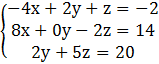### Sample Problem

Is (5/2, 5/2, 3) a solution to this system?#### Solution

Plug in 5/2 for x, 5/2 for y and 3 for z in each equation.

-4(5/2)+2(5/2)+3=-2

-10+5+3=-2

-2=-2 Yes! The solution checks.

8(5/2)-2(3)=14

20-6=14

14=14 Yes! The solution checks.

2(5/2)+5(3)=20

5+15=20

20=20 Yes! The solution checks.

Since the point (5/2, 5/2, 3) fulfills all three equations, it is a solution to the system of equations.